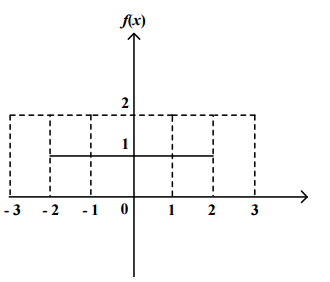### CAT 2000 Question Paper Question 120

Instructions

Directions for the next 3 questions:

Given below are three graphs made up of straight-line segments shown as thick lines. In each case choose the answer as:

a) if f(x)=3f(-x)

b) if f(x)= -f(-x)

c) if f(x) = f(-x)

d) if 3f(x) = 6f(-x), for x >= 0

Question 120

#Solution

In the given graph, the value of y is constant irrespective of the value of x. Hence value of y would be same for a particular x and -x.

Hence f(x) = f(-x)